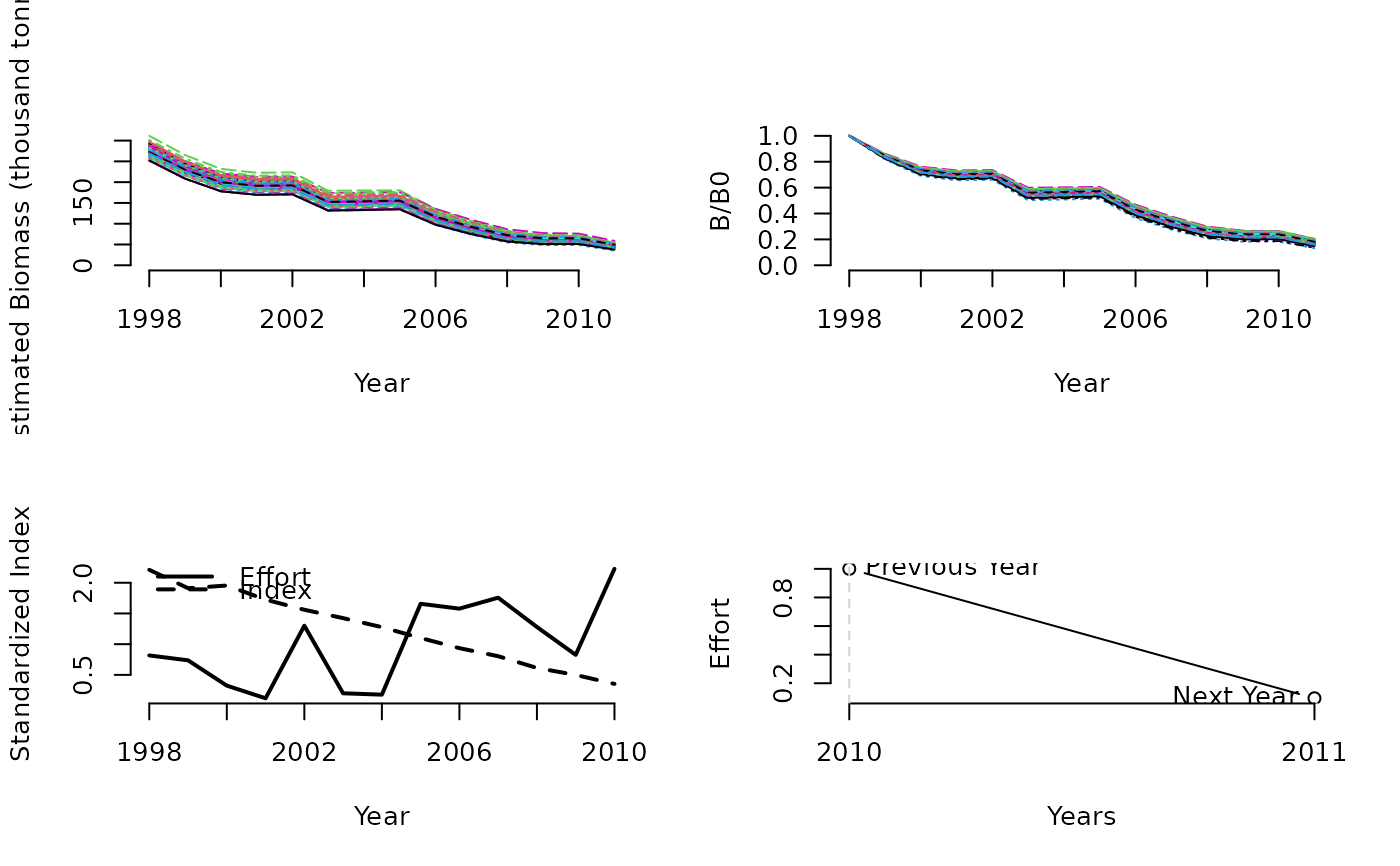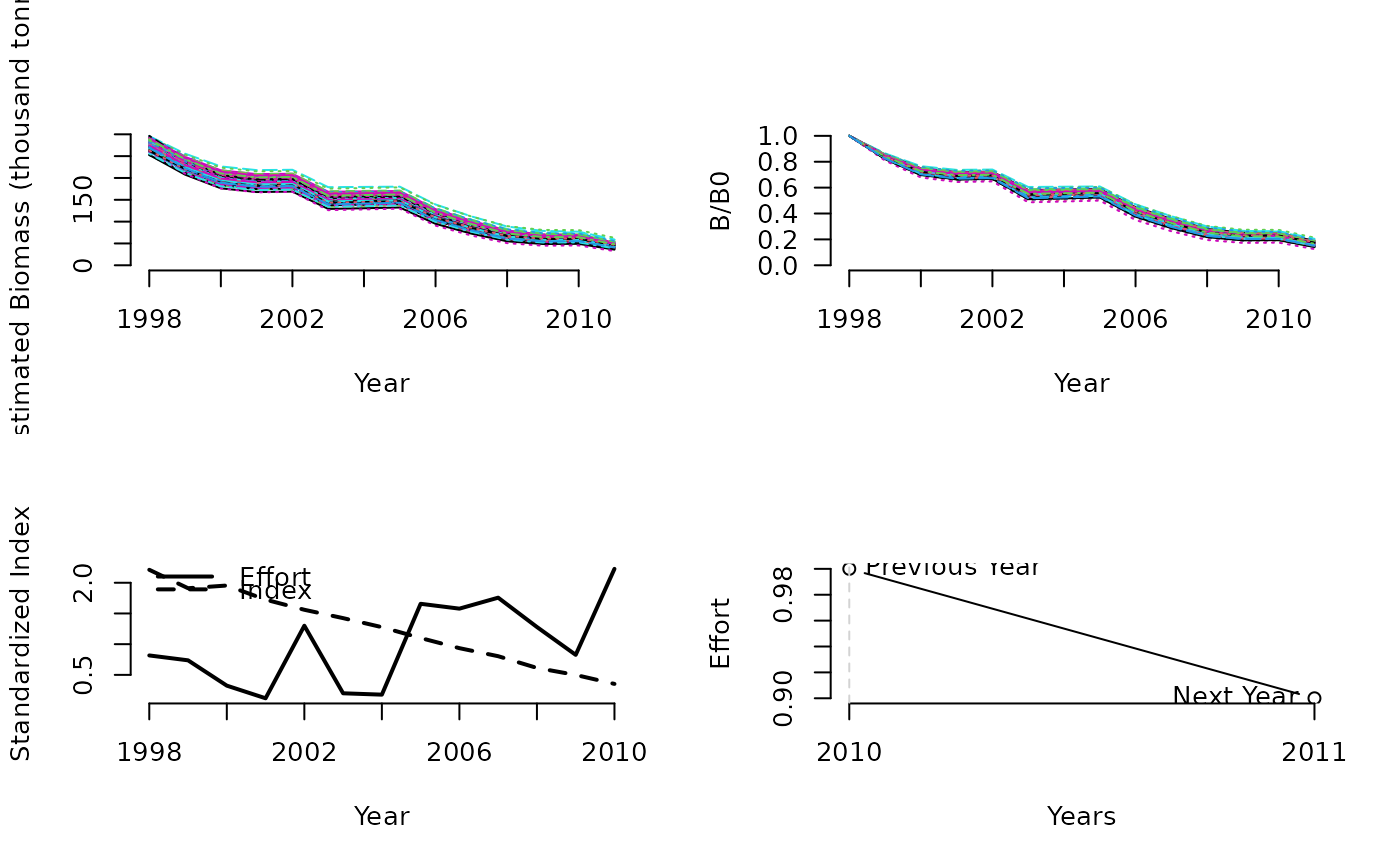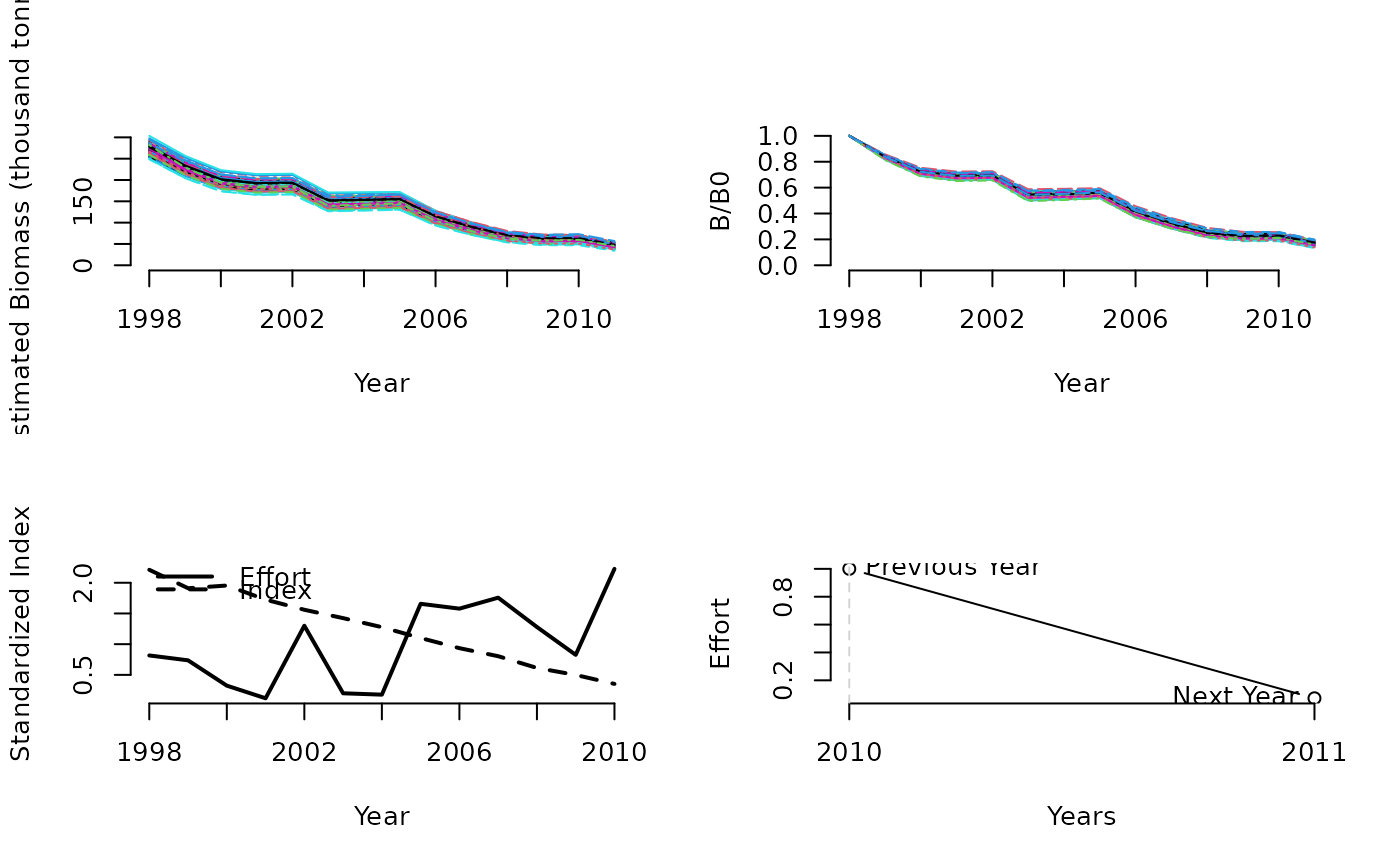A simple delay-difference assessment with UMSY and MSY as leading parameters that estimates $$E_{\textrm{MSY}}$$ using a time-series of catches and a relative abundance index.

DDe(x, Data, reps = 100, plot = FALSE)

DDes(x, Data, reps = 100, plot = FALSE, LB = 0.9, UB = 1.1)

DDe75(x, Data, reps = 100, plot = FALSE)

## Arguments

x

A position in the data object

Data

A data object

reps

The number of stochastic samples of the MP recommendation(s)

plot

Logical. Show the plot?

LB

The lowest permitted factor of previous fishing effort

UB

The highest permitted factor of previous fishing effort

## Value

An object of class Rec-class with the TAE slot(s) populated

## Details

This DD model is observation error only and has does not estimate process error (recruitment deviations). Assumption is that knife-edge selectivity occurs at the age of 50% maturity. Similar to many other assessment models it depends on a whole host of dubious assumptions such as temporally stationary productivity and proportionality between the abundance index and real abundance. Unsurprisingly the extent to which these assumptions are violated tends to be the biggest driver of performance for this method.

The method is conditioned on effort and estimates catch. The effort is calculated as the ratio of catch and index. Thus, to get a complete effort time series, a full time series of catch and index is also needed. Missing values are linearly interpolated.

A detailed description of the delay-difference model can be found in Chapter 9 of Hilborn and Walters (1992).

## Functions

• DDe: Effort-control version. The recommended effort is EMSY.

• DDes: Variant of DDe that limits the maximum change in effort to 10 percent.

• DDe75: Variant of DDe where the recommended effort is 75\

## Required Data

See Data-class for information on the Data object

DDe: Cat, Ind, L50, MPeff, MaxAge, Mort, vbK, vbLinf, vbt0, wla, wlb

## Rendered Equations

See Online Documentation for correctly rendered equations

Other Delay-Difference MPs: DD()

## Examples

DDe(1, Data=MSEtool::Atlantic_mackerel, plot=TRUE)#>     Effort
#> 0.09489645
DDes(1, Data=MSEtool::Atlantic_mackerel, plot=TRUE)#> Effort
#>    0.9
DDe75(1, Data=MSEtool::Atlantic_mackerel, plot=TRUE)#>     Effort
#> 0.07117234